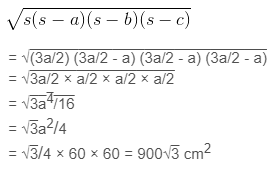Guru

# A traffic signal board, indicating ‘SCHOOL AHEAD’, is an equilateral triangle with side ‘a’. Find the area of the signal board, using Heron’s formula. If its perimeter is 180 cm, what will be the area of the signal board? Q.1

• 0

What is the best way for solving the question from class 9th ncert math of Heron’s Formula chapter of Ncert of exercise 12.1 of math. What is the best way for solving this question please guide me the best way for solving this question. A traffic signal board, indicating ‘SCHOOL AHEAD’, is an equilateral triangle with side ‘a’. Find the area of the signal board, using Heron’s formula. If its perimeter is 180 cm, what will be the area of the signal board?

Share

1. Given,

Side of the signal board = a

Perimeter of the signal board = 3a = 180 cm

∴ a = 60 cm

Semi perimeter of the signal board (s) = 3a/2

By using Heron’s formula,

Area of the triangular signal board will be =• 0